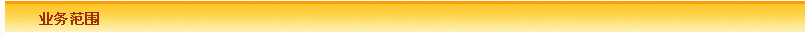9600*1.5%=144元。144*7%=10.08元。144*3%=4.32元。144*2%=2.88元。9600*4%=384元。9600*0.08%=7.68元。例年租金3000*12=36000元。3000*1.5%=45元。45*7%=3.15元。45*3%=1.35元。3000*4%=120元。3000*0.08%=2.4元。*10%=202.72元。例年租金6000*12=72000元。6000*1.5%=90元。6000*4%=240元。6000*0.08%=4.8元。*80%*10%=452.35元。452.35*12=5428.23元。综合税率为13.30%。3000*12=36000元。3000*5%=150元。150*7%=10.5元。150*3%=4.5元。3000*12%=360元。3000*0.1%=3元。*12=533.4*12=6400.8元。*20%=333.32元。333.32*12=3999.84元。6000*5%=300元。6000*12%=720元。6000*0.1%=6元。*80%*20%=789.31元。789.31*12=9471.72元。问 上海高新区公司房产税如何征收？

答 企业租赁房屋作为办公用，需要向房东索要房租发票，当房东不能自己开具房租发票时，公司应去房屋所属的地税所代开房租发票，这时就要缴纳税款。根据上海高新区各个税务所的情况不同，有以下计算方式，科技路地税所、高新路地税所、锦业路地税所、新型工业园地税所征收情况

1、收款方为个人，房屋用途为住宅、公寓、成套住宅

1）月租金800以下 例年租金 800*12=9600元

计税方法 营业税 9600*1.5%=144元

城建税 144*7%=10.08元

教育附加 144*3%=4.32元

地方教育附加 144*2%=2.88元

房产税 9600*4%=384元

水利基金 9600*0.08%=7.68元

合计税款 144+10.08+4.32+2.88+384+7.68=552.96元

综合税率为5.76%

2）月租金4000或4000以下 例年租金3000*12=36000元

计税方法 每月 营业税 3000*1.5%=45元

城建税 45*7%=3.15元

教育附加 45*3%=1.35元

地方教育附加 45*2%=0.9元

房产税 3000*4%=120元

水利基金 3000*0.08%=2.4元

年 （45+3.15+1.35+0.9+120+2.4）*12=172.8*12=2073.6元

个人所得税 月 （3000-172.8-800）*10%=202.72元

年 202.72*12=2432.64元

合计税款 2073.6+2432.64=4506.24元

综合税率为12.52%

3）月租金4000以上 例年租金6000*12=72000元

计税方法 每月 营业税 6000*1.5%=90元

城建税 90*7%=6.3元

教育附加 90*3%=2.7元

地方教育附加 90*2%=1.8元

房产税 6000*4%=240元

水利基金 6000*0.08%=4.8元

年 （90+6.3+2.7+1.8+240+4.8）*12=345.6*12=4147.2元

个人所得税 月 （6000-345.6）*80%*10%=452.35元

年 452.35*12=5428.23元

合计税款 4147.2+5428.23=9575.43元

综合税率为13.30%

2、收款方为个人，房屋用途为商业、办公、商住

1）月租金4000或4000以下 例年租金 3000*12=36000元

计税方法 每月 营业税 3000*5%=150元

城建税 150*7%=10.5元

教育附加 150*3%=4.5元

地方教育附加 150*2%=3元

房产税 3000*12%=360元

水利基金 3000*0.08%=2.4元

印花税 3000*0.1%=3元

年 （150+10.5+4.5+3+360+2.4+3）*12=533.4*12=6400.8元

个人所得税 月 （3000-533.4-800）*20%=333.32元

年 333.32*12=3999.84元

合计税款 6400.8+3999.84=10400.64元

综合税率为28.89%

2）月租金4000以上 例年租金6000*12=72000元

计税方法 每月 营业税 6000*5%=300元

城建税 300*7%=21元

教育附加 300*3%=9元

地方教育附加 300*2%=6元

房产税 6000*12%=720元

水利基金 6000*0.08%=4.8元

印花税 6000*0.1%=6元

年 （300+21+9+6+720+4.8+6）*12=1066.8*12=12801.6元

个人所得税 月 （6000-1066.8）*80%*20%=789.31元

年 789.31*12=9471.72元

合计税款 12801.6+9471.72=22273.32元

综合税率为30.94%

3、收款方为单位

无需缴纳个人所得税，综合税率为17.78%

另外，由于房屋出租金额不一，有高有低，税务局为了核算方便，各个税务所也规定了各自的标准月租金，其中

科技路所旺座国际、旺座现代城标准租金60元/㎡ ，金桥国际50元/㎡ ，甘家寨约25元/㎡ ，其余楼盘最低35元/㎡ ；

高新路所、锦业路所最低35元/㎡；

创业研发园地税所最低40元/㎡